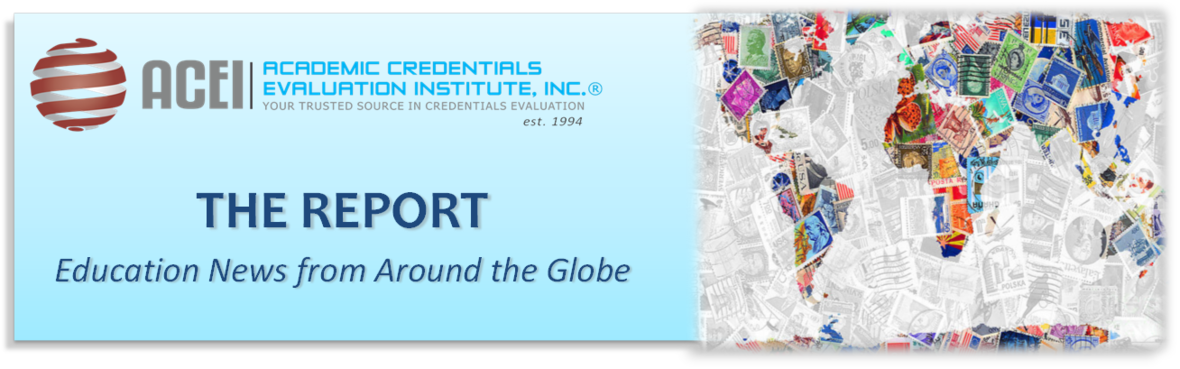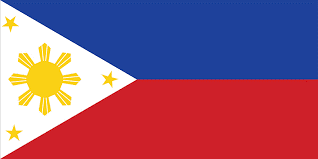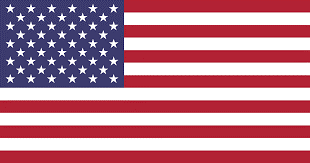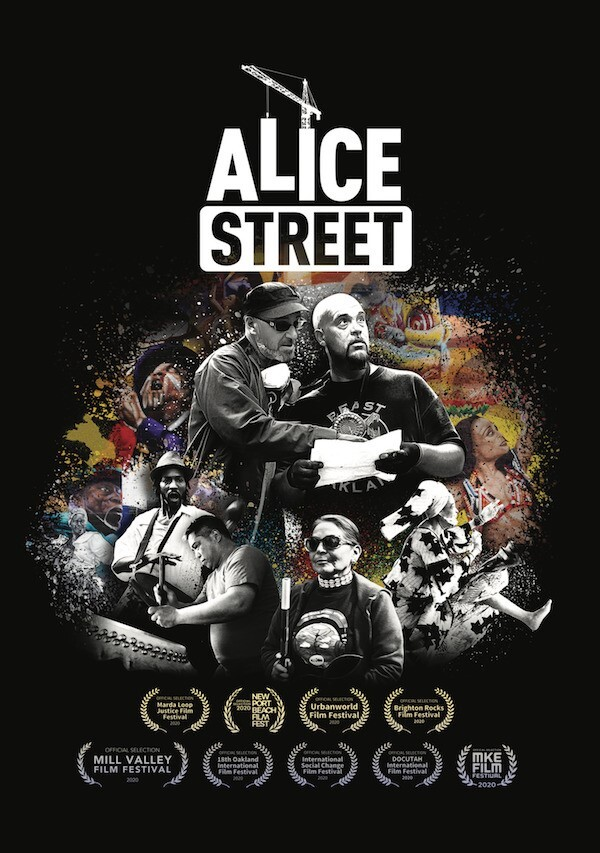# November 2021 - Los Angeles, CA

ACADEMIC CREDENTIALS EVALUATION INSTITUTE, INC. (ACEI)
Founded in 1994, ACEI is a full-service company providing complete and integrated services in-house in the areas of international education research, credential evaluation, and translation. And, through our Global Consulting Group®, we offer our expertise in the following specialties: Media and Branding, Global Pathways, and Diversity, Equity and Inclusion (DEI) to interested institutions and organizations around the globe.

 table div table+table+table div table{width:100%;padding:0}table div table+table+table div table img{width:96.23%;padding:0;float:none}table div table+table+table div table td{width:100%;padding:0 1.88% 18px}/* styles */# The Report Partners

 table div table+table+table+table+table div table{width:100%;padding:0}table div table+table+table+table+table div table table{padding:0;float:left!important;width:28.679%!important}table div table+table+table+table+table div table table+table+table td,table div table+table+table+table+table div table td{padding-left:0;padding-right:0}table div table+table+table+table+table div table table td,table div table+table+table+table+table div table table+table td{padding-left:0;padding-right:20px}table div table+table+table+table+table div table table+table{float:left!important;width:41.698%!important}table div table+table+table+table+table div table table+table+table{float:left!important;width:29.623000000000005%!important}/* styles */
 table div table+table+table+table+table+table div table{width:100%;padding:0}table div table+table+table+table+table+table div table img{width:96.23%;padding:0;float:none}table div table+table+table+table+table+table div table td{width:100%;padding:0 1.88% 18px}/* styles */# UPCOMING EVENTS

 /* styles */ December 8-9, 2021 – IHES Virtual Conference ACEI is a proud sponsor of the International Higher Education for Society (HES) Virtual Conference on December 8-9, 2021. This is a free event. For more information and registration, please click here.
 table div table+table+table+table+table+table+table+table+table div table{width:100%;padding:0}table div table+table+table+table+table+table+table+table+table div table img{width:96.23%;padding:0;float:none}table div table+table+table+table+table+table+table+table+table div table td{width:100%;padding:0 1.88% 18px}/* styles */# ACEI WEBINARS

 table div table+table+table+table+table+table+table+table+table+table+table+table div table{width:100%;padding:0}table div table+table+table+table+table+table+table+table+table+table+table+table div table img{width:96.23%;padding:0;float:none}table div table+table+table+table+table+table+table+table+table+table+table+table div table td{width:100%;padding:0 1.88% 18px}/* styles */# Education News From Around the Globe# Offering Scholarships to International Students

The Flemish Government has announced that it will put aside €2 million every year for scholarships for students in the region who have been accepted into renowned universities abroad. To read more, click here.

 table div table+table+table+table+table+table+table+table+table+table+table+table+table+table+table div table{width:100%;padding:0}table div table+table+table+table+table+table+table+table+table+table+table+table+table+table+table div table img{width:96.23%;padding:0;float:none}table div table+table+table+table+table+table+table+table+table+table+table+table+table+table+table div table td{width:100%;padding:0 1.88% 18px}/* styles */# Only Vaccinated Can Sit for University Examinations

The Minister of Higher Education and Scientific Research Khaled Abdel-Ghaffar announced on Monday, November 23, 2021 that all university students will be required to be vaccinated in order to attend exams at the end of the current academic year’s first semester. To read more, click here.

 table div table+table+table+table+table+table+table+table+table+table+table+table+table+table+table+table+table div table{width:100%;padding:0}table div table+table+table+table+table+table+table+table+table+table+table+table+table+table+table+table+table div table img{width:96.23%;padding:0;float:none}table div table+table+table+table+table+table+table+table+table+table+table+table+table+table+table+table+table div table td{width:100%;padding:0 1.88% 18px}/* styles */# Overhaul in Store for Higher Education

 table div table+table+table+table+table+table+table+table+table+table+table+table+table+table+table+table+table+table+table div table{width:100%;padding:0}table div table+table+table+table+table+table+table+table+table+table+table+table+table+table+table+table+table+table+table div table img{width:96.23%;padding:0;float:none}table div table+table+table+table+table+table+table+table+table+table+table+table+table+table+table+table+table+table+table div table td{width:100%;padding:0 1.88% 18px}/* styles */# New Platform Opens Indian Universities to Ugandans

Makerere University has made it possible for students wishing to pursue their degree courses in universities in India to do so from here through e-learning. To read more, click here.

 table div table+table+table+table+table+table+table+table+table+table+table+table+table+table+table+table+table+table+table+table+table div table{width:100%;padding:0}table div table+table+table+table+table+table+table+table+table+table+table+table+table+table+table+table+table+table+table+table+table div table img{width:96.23%;padding:0;float:none}table div table+table+table+table+table+table+table+table+table+table+table+table+table+table+table+table+table+table+table+table+table div table td{width:100%;padding:0 1.88% 18px}/* styles */# Nigeria's Exams Body, JAMB Uncovers 706,189 Illegal Admissions by Federal, State Universities

The Joint Admissions and Matriculation Board (JAMB) has detected 706,189 illegal admissions by universities, Colleges of Education, polytechnics and other tertiary education institutions. To read more, click here.

 table div table+table+table+table+table+table+table+table+table+table+table+table+table+table+table+table+table+table+table+table+table+table+table div table{width:100%;padding:0}table div table+table+table+table+table+table+table+table+table+table+table+table+table+table+table+table+table+table+table+table+table+table+table div table img{width:96.23%;padding:0;float:none}table div table+table+table+table+table+table+table+table+table+table+table+table+table+table+table+table+table+table+table+table+table+table+table div table td{width:100%;padding:0 1.88% 18px}/* styles */# Replacing Students' Marks with Grades

The Minister for Higher Education, Punjab, Inter Board Committee of Chairmen and Federal Board of Education are unanimous to revamp the grading system in the country to discourage the race of marks. The new grading system will help students know about their progress in the area of cognitive, affective, and psychomotor domains. The students may be provided comprehensive feedback mentioning their weak and strong areas. To read more, click here.

 table div table+table+table+table+table+table+table+table+table+table+table+table+table+table+table+table+table+table+table+table+table+table+table+table+table div table{width:100%;padding:0}table div table+table+table+table+table+table+table+table+table+table+table+table+table+table+table+table+table+table+table+table+table+table+table+table+table div table img{width:96.23%;padding:0;float:none}table div table+table+table+table+table+table+table+table+table+table+table+table+table+table+table+table+table+table+table+table+table+table+table+table+table div table td{width:100%;padding:0 1.88% 18px}/* styles */# Funding for Research Doubles

A recent study by the Department of Science and Technology, the Philippines Statistics Authority and the University of the Philippines Los Baños shows there is a record-breaking increase in human and financial resources supporting research and development (R&D) from 2015 to 2018. To read more, click here.

 table div table+table+table+table+table+table+table+table+table+table+table+table+table+table+table+table+table+table+table+table+table+table+table+table+table+table+table div table{width:100%;padding:0}table div table+table+table+table+table+table+table+table+table+table+table+table+table+table+table+table+table+table+table+table+table+table+table+table+table+table+table div table img{width:96.23%;padding:0;float:none}table div table+table+table+table+table+table+table+table+table+table+table+table+table+table+table+table+table+table+table+table+table+table+table+table+table+table+table div table td{width:100%;padding:0 1.88% 18px}/* styles */# Audits in Progress to Determine Cheating During Online Learning

The Council on Higher Education (CHE) is conducting institutional audits at universities. This comes after a survey at tertiary institutions about perspectives on remote learning and teaching raised concerns about cheating. To read more, click here.

 table div table+table+table+table+table+table+table+table+table+table+table+table+table+table+table+table+table+table+table+table+table+table+table+table+table+table+table+table+table div table{width:100%;padding:0}table div table+table+table+table+table+table+table+table+table+table+table+table+table+table+table+table+table+table+table+table+table+table+table+table+table+table+table+table+table div table img{width:96.23%;padding:0;float:none}table div table+table+table+table+table+table+table+table+table+table+table+table+table+table+table+table+table+table+table+table+table+table+table+table+table+table+table+table+table div table td{width:100%;padding:0 1.88% 18px}/* styles */# A New Push to Create a 3-Year Degree Option

Higher education thought leaders and colleges are working together to create a three-year bachelor’s degree program that will offer all the value of a four-year degree—for less cost. To read more, click here.

 table div table+table+table+table+table+table+table+table+table+table+table+table+table+table+table+table+table+table+table+table+table+table+table+table+table+table+table+table+table+table+table div table{width:100%;padding:0}table div table+table+table+table+table+table+table+table+table+table+table+table+table+table+table+table+table+table+table+table+table+table+table+table+table+table+table+table+table+table+table div table img{width:96.23%;padding:0;float:none}table div table+table+table+table+table+table+table+table+table+table+table+table+table+table+table+table+table+table+table+table+table+table+table+table+table+table+table+table+table+table+table div table td{width:100%;padding:0 1.88% 18px}/* styles */# FILMS & DOCUMENTARIES

 /* styles */ For your viewing pleasure, we’ve selected the following documentaries, available through our partner Video Project:/* styles */ In a rapidly gentrifying city, the construction of a luxury condominium threatens a local mural, forcing the artists and neighborhood to rally to protect its history, voice, and land. Two artists — Chilean studio painter Pancho Peskador and Chicago-born aerosol artist Desi Mundo — form an unlikely partnership to paint their most ambitious mural to date in downtown Oakland. The four-story mural is situated at a unique intersection of Chinese and Afro-Diasporic communities who face the imminent threat of displacement and gentrification. But even before painting can commence, the artists face numerous obstacles such as complex negotiations with profit minded property owners, satisfying a community of diverse residents, and resolving the artists' own aesthetic conflicts. For more information, click here.
 table div table+table+table+table+table+table+table+table+table+table+table+table+table+table+table+table+table+table+table+table+table+table+table+table+table+table+table+table+table+table+table+table+table+table+table+table div table{width:100%;padding:0}table div table+table+table+table+table+table+table+table+table+table+table+table+table+table+table+table+table+table+table+table+table+table+table+table+table+table+table+table+table+table+table+table+table+table+table+table div table img{width:96.23%;padding:0;float:none}table div table+table+table+table+table+table+table+table+table+table+table+table+table+table+table+table+table+table+table+table+table+table+table+table+table+table+table+table+table+table+table+table+table+table+table+table div table td{width:100%;padding:0 1.88% 18px}/* styles */table.module-37{width:81.7%;padding:0}table div table+table+table+table+table+table+table+table+table+table+table+table+table+table+table+table+table+table+table+table+table+table+table+table+table+table+table+table+table+table+table+table+table+table+table+table+table+table div table{width:81.7%;float:none;margin-left:auto;margin-right:auto;padding:0}table div table+table+table+table+table+table+table+table+table+table+table+table+table+table+table+table+table+table+table+table+table+table+table+table+table+table+table+table+table+table+table+table+table+table+table+table+table+table div table a{border:0 none;text-decoration:none}table div table+table+table+table+table+table+table+table+table+table+table+table+table+table+table+table+table+table+table+table+table+table+table+table+table+table+table+table+table+table+table+table+table+table+table+table+table+table div table img{width:100%!important;border:0 none;text-decoration:none}table div table+table+table+table+table+table+table+table+table+table+table+table+table+table+table+table+table+table+table+table+table+table+table+table+table+table+table+table+table+table+table+table+table+table+table+table+table+table div table td{width:100%;padding:0}/* styles */

## ACEI Global Consulting Group

The Global Consulting Group is a division of ACEI that provides international education expertise with focus on Diversity, Equity, Inclusion Consulting, Global Pathway Consulting (Program/Curriculum Review for Partnerships between overseas institutions), and Media and Brand Consulting. Click here to view video presentation.

 table div table+table+table+table+table+table+table+table+table+table+table+table+table+table+table+table+table+table+table+table+table+table+table+table+table+table+table+table+table+table+table+table+table+table+table+table+table+table+table+table div table{width:100%;padding:0}table div table+table+table+table+table+table+table+table+table+table+table+table+table+table+table+table+table+table+table+table+table+table+table+table+table+table+table+table+table+table+table+table+table+table+table+table+table+table+table+table div table img{width:96.23%;padding:0;float:none}table div table+table+table+table+table+table+table+table+table+table+table+table+table+table+table+table+table+table+table+table+table+table+table+table+table+table+table+table+table+table+table+table+table+table+table+table+table+table+table+table div table td{width:100%;padding:0 1.88% 18px}/* styles *//* styles */ ACEI offers a comprehensive range of professional services that include: international credential evaluations; translations; consulting; and training. For more information on ACEI, please visit www.acei-global.org or contact: Tel: 1-310-275-3530; Email: acei@acei-global.org. ACEI was founded and incorporated in 1994 in the State of California and is a Charter and Endorsed Member of the Association of International Credential Evaluators (AICE).
 table div table+table+table+table+table+table+table+table+table+table+table+table+table+table+table+table+table+table+table+table+table+table+table+table+table+table+table+table+table+table+table+table+table+table+table+table+table+table+table+table+table+table div table{width:100%;padding:0}table div table+table+table+table+table+table+table+table+table+table+table+table+table+table+table+table+table+table+table+table+table+table+table+table+table+table+table+table+table+table+table+table+table+table+table+table+table+table+table+table+table+table div table img{width:96.23%;padding:0;float:none}table div table+table+table+table+table+table+table+table+table+table+table+table+table+table+table+table+table+table+table+table+table+table+table+table+table+table+table+table+table+table+table+table+table+table+table+table+table+table+table+table+table+table div table td{width:100%;padding:0 1.88% 18px}/* styles */# New to The Report? Click below to subscribe to this newsletter.

 Like   Tweet   Pin   +1   in